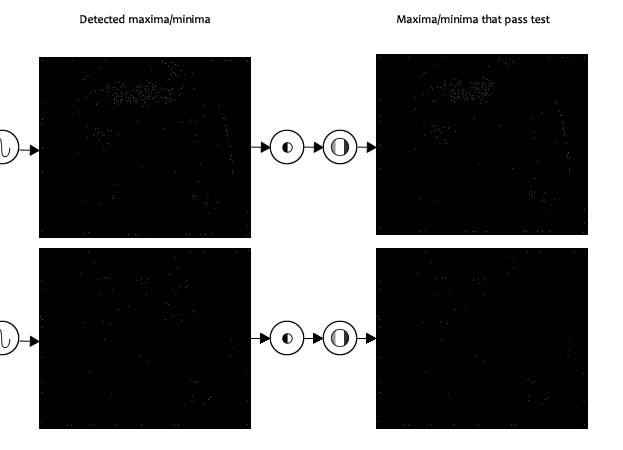## Getting rid of low contrast keypoints

Series: SIFT: Theory and Practice:

1. Introduction
2. The scale space
3. LoG approximations
4. Finding keypoints
5. Getting rid of low contrast keypoints
6. Keypoint orientations
7. Generating a feature

Key points generated in the previous step produce a lot of key points. Some of them lie along an edge, or they don't have enough contrast. In both cases, they are not useful as features. So we get rid of them. The approach is similar to the one used in the Harris Corner Detector for removing edge features. For low contrast features, we simply check their intensities.

## Removing low contrast features

This is simple. If the magnitude of the intensity (i.e., without sign) at the current pixel in the DoG image (that is being checked for minima/maxima) is less than a certain value, it is rejected.

Because we have subpixel keypoints (we used the Taylor expansion to refine keypoints), we again need to use the taylor expansion to get the intensity value at subpixel locations. If it's magnitude is less than a certain value, we reject the keypoint.

## Removing edges

The idea is to calculate two gradients at the keypoint. Both perpendicular to each other. Based on the image around the keypoint, three possibilities exist. The image around the keypoint can be:

• A flat region: If this is the case, both gradients will be small.
• An edge: Here, one gradient will be big (perpendicular to the edge) and the other will be small (along the edge)
• A "corner": Here, both gradients will be big.

Corners are great keypoints. So we want just corners. If both gradients are big enough, we let it pass as a key point. Otherwise, it is rejected.

Mathematically, this is achieved by the Hessian Matrix. Using this matrix, you can easily check if a point is a corner or not.

If you're interested in the math, first check the posts on the Harris corner detector. A lot of the same math used used in SIFT. In the Harris Corner Detector, two eigenvalues are calculated. In SIFT, efficiency is increased by just calculating the ratio of these two eigenvalues. You never need to calculate the actual eigenvalues.

## Example

Here's a visual example of what happens in this step:Both extrema images go through the two tests: the contrast test and the edge test. They reject a few keypoints (sometimes a lot) and thus, we're left with a lower number of keypoints to deal with.

## Summary

In this step, the number of keypoints was reduced. This helps increase efficiency and also the robustness of the algorithm. Keypoints are rejected if they had a low contrast or if they were located on an edge.

In the next step we'll assign an orientation to all the keypoints that passed both tests.

## More in the series

This tutorial is part of a series called SIFT: Theory and Practice:

1. Introduction
2. The scale space
3. LoG approximations
4. Finding keypoints
5. Getting rid of low contrast keypoints
6. Keypoint orientations
7. Generating a feature

Utkarsh Sinha created AI Shack in 2010 and has since been working on computer vision and related fields. He is currently at Microsoft working on computer vision.